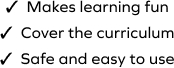# Calculate the Change Received in the Given Word Problems

SplashLearn - The complete PreK - Grade 5 Math & ELA Learning Program Built for Your Child
Home > Calculate the Change Received in the Given Word Problems
The game requires students to complete a set of challenging tasks and the students practice more on the concepts of counting money in the process. Students will strengthen their problem-solving ability by working with subtraction word problems in this game. They will work on a set of change unknown scenarios and use subtraction to find the final answer. Students will choose the correct answer from the given options to solve the problems.